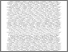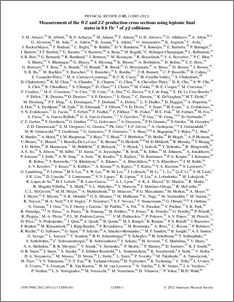# A measurement of the WZ and ZZ production cross sections using leptonic final states in 8.6 fb$^{-1}$ of $p\bar{p}$ collisions

Collaboration, D0 and Bertram, Iain and Borissov, Guennadi and Fox, Harald and Ross, Anthony and Williams, Mark and Ratoff, Peter (2012) A measurement of the WZ and ZZ production cross sections using leptonic final states in 8.6 fb$^{-1}$ of $p\bar{p}$ collisions. Physical Review D, 85 (11). ISSN 1550-7998Preview
PDF
PhysRevD.85.112005.pdf - Published Version

## Abstract

We study the processes $p\bar{p} \rightarrow WZ \rightarrow \ell\nu\ell^+\ell-$ and $p\bar{p} \rightarrow ZZ \rightarrow \ell^+\ell^-\nu\bar{\nu}, where$\ell$=$e$or${\mu}$. Using 8.6 fb$^{-1} of integrated luminosity collected by the D0 experiment at the Fermilab Tevatron collider, we measure the $WZ$ production cross section to be 4.50$^{+0.63}_{-0.66} pb which is consistent with, but slightly above a prediction of the standard model. The ZZ cross section is measured to be 1.64$\pm$0.46 pb, in agreement with a prediction of the standard model. Combination with an earlier analysis of the$ZZ \rightarrow \ell^+\ell^-\ell^+\ell^-$channel yields a$ZZ$cross section of 1.44$^{+0.35}_{-0.34}\$ pb.

Item Type:
Journal Article
Journal or Publication Title:
Physical Review D